Next: The Newton-Raphson Method Up: Optimization Previous: Method of Steepest Descent

As seen in the previous subsection, the reason why the method of Steepest Descent converges slowly is that it has to take a right angle turn after each step, and consequently search in the same direction as earlier steps (see Figure 4.1). The method of Conjugate Gradients is an attempt to mend this problem by ``learning'' from experience.

``Conjugacy'' means that two unequal vectors,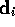and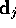, are orthogonal with respect to any symmetric positive definite matrix, for example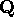in (4.6); i.e.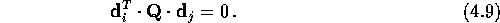This can be looked upon as a generalization of orthogonality, for whichis the unity matrix. The idea is to let each search directionbe dependent on all the other directions searched to locate the minimum ofthrough equation (4.9). A set of such search directions is referred to as a-orthogonal, or conjugate, set, and it will take a positive definite n-dimensional quadratic function as given in (4.6) to its minimum point in, at most, n exact linear searches. This method is often referred to as Conjugate Directions, and a short description follows.

The best way to visualize the working of Conjugate Directions is by comparing the space we are working in with a ``stretched'' space. An example of this ``stretching'' of space is illustrated in Figure 4.3: (a) demonstrates the shape of the contours of a quadratic function in real space, which are elliptical (for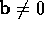). Any pair of vectors that appear perpendicular in this space, would be orthogonal. (b) show the same drawing in a space that are stretched along the eigenvectoraxes so that the elliptical contours from (a) become circular. Any pair of vectors that appear to be perpendicular in this space, is in fact-orthogonal. The search for a minimum of the quadratic functions starts at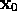in Figure 4.3(a), and takes a step in the directionand stops at the point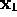. This is a minimum point along that direction, determined the same way as for the Steepest Decent method in the previous section: the minimum along a line is where the directional derivative is zero (equation (4.8)). The essential difference between the Steepest Descent and the Conjugate Directions lies in the choice of the next search direction from this minimum point. While the Steepest Descent method would search in the direction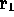in Figure 4.3(a), the Conjugate Direction method would chose. How come Conjugate Directions manage to search in the direction that leads us straight to the solution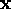? The answer is found in Figure 4.3(b): In this stretched space, the directionappears to be a tangent to the now circular contours at the point. Since the next search directionis constrained to be-orthogonal to the previous, they will appear perpendicular in this modified space. Hence,will take us directly to the minimum point of the quadratic function.Figure 4.3: Optimality of the method of Conjugate Directions. (a) Lines that appear perpendicular are orthogonal. (b) The same problem in a ``stretched'' space. Lines that appear perpendicular are-orthogonal.

To avoid searching in directions that have been searched before, the Conjugate Direction guarantees that the minimization ofalong one direction does not ``spoil'' the minimization along another; i.e. that after i steps,will be minimized over all searched directions. This is essentially what is stated in equation (4.9): A search alonghas revealed where the gradient is perpendicular to,, and as we now move along some new direction, the gradient changes by(see equation (4.6)). In order to not interfere with the minimization along, we require that the gradient remain perpendicular to; i.e. that the change in gradient itself be perpendicular to. Hence, we have equation (4.9). We see from Figure 4.3(b), whereandappear perpendicular because they are-orthogonal, that it is clear thatmust point to the solution. A thorough description of the linear Conjugate Directions and Conjugate Gradients methods has been given by Shew.

The Conjugate Gradients method is a special case of the method of Conjugate Directions, where the conjugate set is generated by the gradient vectors. This seems to be a sensible choice since the gradient vectors have proved their applicability in the Steepest Descent method, and they are orthogonal to the previous search direction. For a quadratic function, as given in equation (4.6), the procedure is as follows.

The initial step is in the direction of the steepest descent:Subsequently, the mutually conjugate directions are chosen so that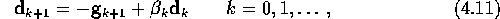where the coefficientis given by, for example, the so called Fletcher-Reeves formula: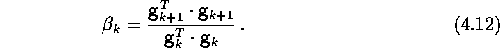For details, see Walsh. The step length along each direction is given by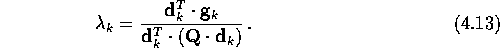The resulting iterative formula is identical to (4.5).

When the matrixin (4.13) is not known, or is too computationally costly to determine, the stepsize can be found by linear searches. For the Conjugate Gradient method to converge in n iterations, these linear searches need to be accurate. Even small deviations can cause the search vectors to lose-orthogonality, resulting in the method spending more than n iterations in locating the minimum point. In practice, accurate linear searches are impossible, both due to numerical accuracy and limited computing time. The direct use of equation (4.13) will most likely not bring us to the solution in n iterations either, the reason being the limited numerical accuracy in the computations which gradually will make the search vectors lose their conjugacy. It should also be mentioned that if the matrixis badly scaled, the convergence will be slowed down considerably, as it was for the Steepest Descent method.

Even though the Conjugate Gradients method is designed to find the minimum point for simple quadratic functions, it also does the job well for any continuous functionfor which the gradient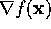can be computed. Equation (4.13) can not be used for non-quadratic functions, so the step length has to be determined by linear searches. The conjugacy of the generated directions may then progressively be lost, not only due to the inaccurate linear searches, but also due to the non-quadratic terms of the functions. An alternative to the Fletcher-Reeves formula that, to a certain extent, deals with this problem, is the Polak-Ribière formula Press:The difference in performance between these two formulas is not big though, but the Polak-Ribière formula is known to perform better for non-quadratic functions.

The iterative algorithm for the Conjugate Gradients method for non-quadratic functions using the Polak-Ribière formula is given in Algorithm 4.2.Regardless of the direction-update formula used, one must deal with the loss of conjugacy that results from the the non-quadratic terms. The Conjugate Gradients method is often employed to problems where the number of variables n is large, and it is not unusual for the method to start generating nonsensical and inefficient directions of search after a few iterations. For this reason it is important to operate the method in cycles, with the first step being the Steepest Descent step. One example of a restarting policy is to restart with the Steepest Descent step after n iterations after the preceding restart.

Another practical issue relates to the accuracy of the linear search that is necessary for efficient computation. On one hand, an accurate linear search is needed to limit the loss of direction conjugacy. On the other hand, insisting on very accurate line search can be computationally expensive. To find the best possible middle path, trial and error is needed.

The Conjugate Gradients method is apart from being an optimization method, also one of the most prominent iterative method for solving sparse systems of linear equations. It is fast and uses small amounts of storage since it only needs the calculation and storage of the second derivative at each iteration. The latter becomes significant when n is so large that problems of computer storage arises. But, everything is not shiny; the less similar f is to a quadratic function, the more quickly the search directions lose conjugacy, and the method may in the worst case not converge at all. Although, it is generally to be preferred over the Steepest Descent method.Next: The Newton-Raphson Method Up: Optimization Previous: Method of Steepest Descent

Trond Hjorteland
Mon Jul 5 02:59:28 MET DST 1999